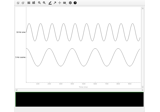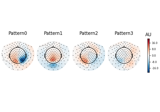# mne.time_frequency.psd_array_welch#

mne.time_frequency.psd_array_welch(x, sfreq, fmin=0, fmax=inf, n_fft=256, n_overlap=0, n_per_seg=None, n_jobs=None, average='mean', window='hamming', remove_dc=True, *, output='power', verbose=None)[source]#

Compute power spectral density (PSD) using Welch’s method.

Welch’s method is described in Welch.

Parameters:
x`array`, shape=(…, n_times)

The data to compute PSD from.

sfreq`float`

The sampling frequency.

fmin`float`

The lower frequency of interest.

fmax`float`

The upper frequency of interest.

n_fft`int`

The length of FFT used, must be `>= n_per_seg` (default: 256). The segments will be zero-padded if `n_fft > n_per_seg`.

n_overlap`int`

The number of points of overlap between segments. Will be adjusted to be <= n_per_seg. The default value is 0.

n_per_seg

Length of each Welch segment (windowed with a Hamming window). Defaults to None, which sets n_per_seg equal to n_fft.

n_jobs

The number of jobs to run in parallel. If `-1`, it is set to the number of CPU cores. Requires the `joblib` package. `None` (default) is a marker for ‘unset’ that will be interpreted as `n_jobs=1` (sequential execution) unless the call is performed under a `joblib.parallel_config` context manager that sets another value for `n_jobs`.

average

How to average the segments. If `mean` (default), calculate the arithmetic mean. If `median`, calculate the median, corrected for its bias relative to the mean. If `None`, returns the unaggregated segments.

New in v0.19.0.

window

Windowing function to use. See `scipy.signal.get_window()`.

New in v0.22.0.

remove_dc`bool`

If `True`, the mean is subtracted from each segment before computing its spectrum.

output`str`

The format of the returned `psds` array, `'complex'` or `'power'`:

• `'power'` : the power spectral density is returned.

• `'complex'` : the complex fourier coefficients are returned per window.

New in v1.4.0.

verbose

Control verbosity of the logging output. If `None`, use the default verbosity level. See the logging documentation and `mne.verbose()` for details. Should only be passed as a keyword argument.

Returns:
psds`ndarray`, shape (…, n_freqs) or (…, n_freqs, n_segments)

The power spectral densities. If `average='mean` or `average='median'`, the returned array will have the same shape as the input data plus an additional frequency dimension. If `average=None`, the returned array will have the same shape as the input data plus two additional dimensions corresponding to frequencies and the unaggregated segments, respectively.

freqs`ndarray`, shape (n_freqs,)

The frequencies.

Notes

New in v0.14.0.

References

## Examples using `mne.time_frequency.psd_array_welch`#Creating MNE-Python data structures from scratch

Creating MNE-Python data structures from scratchCompute Spectro-Spatial Decomposition (SSD) spatial filters

Compute Spectro-Spatial Decomposition (SSD) spatial filters